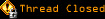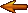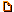# Topic awaiting preservation: Simple Trig. question (Page 1 of 1)Dracusis
Maniac (V) Inmate

From: Brisbane, Australia
Insane since: Apr 2001posted 03-10-2003 00:17

My brain isn't working today so I thought I'd borrow yours.

There should be a rather simple solution to this. Say I have a rectangle, the rectangle will *always* be the same size. What I need to know is weather point x,y inside this rectangle is in diagonal half a or diagonal half b.

For example:
<BLOCKQUOTE><FONT face="Verdana, Arial">code:</font><HR><pre>
-----

Slime

From: Massachusetts, USA
Insane since: Mar 2000posted 03-10-2003 00:27

This math might be able to be simplified in order to reduce the number of operations the processor needs to do:

x = (x-left_edge_coordinate)/(right_edge_coordinate-left_edge_coordinate)
y = (y-bottom_edge_coordinate)/(top_edge_coordinate-bottom_edge_coordinate)
/* (note that the denominators in those terms can be precalculated as the "width" and "height", and you can precalculate their reciprocals to do multiplication intead of division.) */
return x > y // returns true if in bottom triangle, false if in top triangle. this works because x=y is the diagonal line that separates the two triangles.

That should do it.

Dracusis
Maniac (V) Inmate

From: Brisbane, Australia
Insane since: Apr 2001posted 03-10-2003 00:40

Execelent, thanks slime! That works a treat.

Um, is there any performance difference between multiplication and division? Because I can simple pass it the width and height valuse if that'd be faster.

InI

From: Somewhere over the rainbow
Insane since: Mar 2001posted 03-10-2003 00:58

The poster has demanded we remove all his contributions, less he takes legal action.
We have done so.
Now Tyberius Prime expects him to start complaining that we removed his 'free speech' since this message will replace all of his posts, past and future.
Don't follow his example - seek real life help first.

Dracusis
Maniac (V) Inmate

From: Brisbane, Australia
Insane since: Apr 2001posted 03-10-2003 01:23

Ok so this should work right...

x is the point's x value
y is the point's y value
w is the rectangle width
h is the rectangle height

lets assume the rectangle is at 0,0

function pointAorB(x,y,h,w) { //returns true if a, false if b
x = x/w
y = (y-h)/-h
return x < y
}

and if I wanted to change the Diagional to run from top left to bottom right it would simply be an ((x/w) < (y/h)) comparasion right?

[This message has been edited by Dracusis (edited 03-10-2003).]

Slime

From: Massachusetts, USA
Insane since: Mar 2000posted 03-10-2003 04:16

That code you gave will do the test for the diagonal from the upper left to the lower right. To do the test from the lower left to the top right, just change the line

y = (y-h)/-h

to

y = y/h

InI: is there really no difference between division and multiplication? In a scripting language like JavaScript, it would hardly matter anyway. But in real programs where the operation will be done a *lot*, is there no speedup?

Dracusis
Maniac (V) Inmate

From: Brisbane, Australia
Insane since: Apr 2001posted 03-10-2003 04:37

Ay, thanks again slime, I had em the wrong way around. Silly me.

Actually, I'm using this in Director and it's being calculated up to 30 times a second -- faster than JavaScript but slower than Java. I only posted here because it didn't really seem like a "multimedia" question.

Anyways, this is to detect which hexagon the mouse in inside for my game map. I read a bunch of articles on the math involved for detecting mouse clicks in hexes and well, they all seemed stupidly complex for what it was doing. Now I'm just detecting if the mouse falls into the bounding box of each tile. If it falls into two bounding boxes at once then I have to do a further calculation, which is the triangle based one (each triangle is a corner of a hex where two hexes bounding boxes overlap).

This calculation gets done on every frame (30 frames a second). At the moment, this along with the tile drawing functions (which won't be calculated on every frame but I haven't optimised them yet) is eating around 30% (on top of currently running background apps, 60% total) of my CPU time on an Athlon 1.67 Ghz. The target machines for this project are a 1Ghz P4 PC and a 400Mhz G4 Mac which is probably cutting things a little close at the moment. Things should improve a heap once I optimise all of the redundant pixel copying but I still have a bunch of other calculations to work into it and well, it's better to be on the safer side.

So, any performance gains I can squeeze out of this would be a help.

[This message has been edited by Dracusis (edited 03-10-2003).]

Slime

From: Massachusetts, USA
Insane since: Mar 2000posted 03-10-2003 06:12

Here's the function I'd use for ultimate speed:

precalculate (if possible):

hovrw = height/width;
x0,y0 = lower left coordinates of rectangle

function:

function pointAorB(x,y,x0,y0) { //returns true if a, false if b
return (x-x0)*hovrw < (y-y0)
}

That's probably about as fast as it gets.

InIposted 03-10-2003 14:02